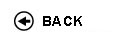Politecnico di Torino
16ACFLZ, 16ACFLN, 16ACFLP, 16ACFLS, 16ACFLX, 16ACFMA, 16ACFMB, 16ACFMC, 16ACFMH, 16ACFMK, 16ACFMN, 16ACFMO, 16ACFMQ, 16ACFNL, 16ACFNM, 16ACFNX, 16ACFOA, 16ACFOD, 16ACFPC, 16ACFPI, 16ACFPL, 16ACFQR
Mathematical analysis I
1st degree and Bachelor-level of the Bologna process in Aerospace Engineering - Torino
1st degree and Bachelor-level of the Bologna process in Automotive Engineering - Torino
1st degree and Bachelor-level of the Bologna process in Electronic And Communications Engineering - Torino
Espandi...
 Teacher Status SSD Les Ex Lab Tut Years teaching Adami Riccardo O2 MAT/05 60 40 0 0 8 Berchio Elvise O2 MAT/05 60 40 0 0 8 Berchio Elvise O2 MAT/05 60 40 0 0 8 Camporesi RobertoAC MAT/05 60 40 0 0 10 Ceragioli Francesca MariaA2 MAT/05 60 40 0 0 10 Ceragioli Francesca MariaA2 MAT/05 60 40 0 0 10 Chiado' Piat ValeriaO2 MAT/05 60 40 0 0 18 Codegone Marco60 40 0 60 14 Grillo Alfio A2 MAT/07 60 40 0 0 8 Mazzi LuisaAC MAT/05 60 40 0 0 21 Nicola Fabio O2 MAT/05 60 40 0 0 10 Pandolfi Luciano60 40 0 0 12 Pandolfi Luciano60 40 0 0 12 Tabacco Anita MariaPO MAT/05 60 40 0 0 22 Tilli PaoloPO MAT/05 60 40 0 0 9 Zanini ChiaraA2 MAT/05 60 40 0 0 7 Zanini ChiaraA2 MAT/05 60 40 0 0 7 Cortese Paolo60 40 0 0 12
 SSD CFU Activities Area context MAT/05 10 A - Di base Matematica, informatica e statistica
Esclusioni:
04KWQ
 Subject fundamentals This course represents a bridge between high school and college ways of teaching and learning. The main goal of the course is to provide the students with tools to understand and elaborate logical arguments, from simple to more elaborate ones, and to introduce the elements of differential and integral calculus for functions of one variable with applications to ordinary differential equations of the first order and of the second order linear case. Expected learning outcomes Ability to follow a chain of logical arguments. Essentials of differential and integral calculus for functions of one variable. Computational ability. Prerequisites / Assumed knowledge Numbers, equations and inequalities, analytical geometry and trigonometry. First properties of elementary functions. Contents Preliminaries: sets and operations with sets. Numerical sets, maxima, minima and extrema. The completeness propertiy of real numbers and its consequences. Functions: surjectivity and injectivity; composition of functions, inverse functions. Functions of a real variable: elementary functions, monotone functions and inverse functions. (About 15 hours) Limits and continuity: theory of limits of functions and sequences. Continuous functions and related theorems. Order and comparison theorems. Algebra of limits. Indeterminate forms. Local comparison of functions. Landau symbols. Infinite and infinitesimal functions. Order of an infinity and of an infinitesimal, principal part with respect to a test function. Asymptotes. The number e. Fundamental trigonometric and exponential limits. Properties continuous functions on an interval: existence of zeros and of maxima and minima. (About 24 hours) Derivatives: geometrical and physical meaning. Computation of derivatives. List of fundamental derivatives. Derivatives and continuity. Lack of differentiability, extremal and critical points. Fermat Theorem. Properties of differentiable functions on an interval and fundamental theorems of differential calculus (Rolle and Lagrange theorems) and their consequences. De L'H�pital rule. Taylor formula and fundamental MacLaurin formulas. Use of Taylor expansions in the local analysis of functions: comparison, extrema and convexity. (About 23 hours) Primitives: rules of computation; primitives of rational functions. Indefinite integral. Riemann integral and its properties. Classes of integrable functions. Integral mean value. Fundamental theorem of integral calculus: relations between definite integrals and primitives. Improper integrals: definitions and convergence tests. (About 21 hours) Complex numbers and differential equations: algebraic and trigonometric forms of complex numbers. Real and imaginary parts, modulus and argument. Roots of complex numbers; Fundamental theorem of Algebra. Exponentials of complex numbers and Euler formulas. Ordinary differential equations: Cauchy problem. First order ordinary differential equations, linear or with separable variables. Second order linear differential equations with constant coefficients. (About 17 hours) Delivery modes 60 hours of theoretical classes and 40 hours of exercise classes. Texts, readings, handouts and other learning resources The following course materials are to be considered as indicative. Further details will be provided by professors in class. C. Canuto, A. Tabacco. Analisi Matematica I. Springer-Verlag Italia, 2014. S. Lancelotti. Lezioni di Analisi Matematica 1. Celid, 2013. F. Nicola. Analisi Matematica I. Appunti delle lezioni. CLUT, 2013. Assessment and grading criteria The exam consists of a test, of a written part and, when required, of an oral part. The test (on a computer, time allowed one hour) consists of 20 multiple-choice questions, including theoretical ones. Each question is worth one mark and the maximum score is 20. If the score is less than 12, then the exam is failed, otherwise the student proceeds with the written part of the exam (exercises and theory, time allowed one hour). The written part has a maximum score of 13 marks. The final score of the exam is the sum of the scores of the test and of the written part, unless a further oral exam is required by the teacher (or by the student, provided the final score is at least 18/30). In this case, the final score will be based also on the oral part. Programma definitivo per l'A.A.2015/16